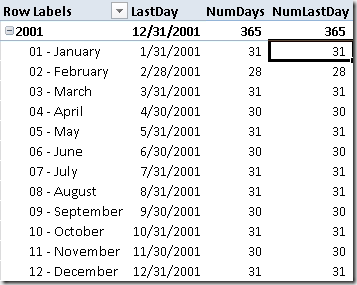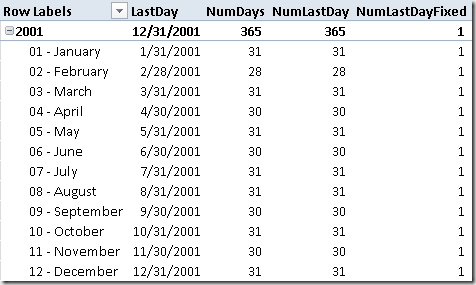This article discusses how to correctly pass the <dates> argument in order to obtain the desired result also in complex expressions.A longer and detailed discussion is available on a blog post written by Jeffrey Wang. From hereinafter we consider just one of the many Time Intelligence functions (LASTDATE), but the same concepts can be applied to all of the Time Intelligence functions that have a <dates> argument.

The LASTDATE function returns the last date in the current context for the specified column of dates. This is the definition that you can find in the documentation, but it might be not obvious how to use it in complex expression, for example when a row context is involved. The syntax for LASTDATE is:

`LASTDATE (  )`

The dates argument is defined as “a column that contains dates”. Many of the examples available refer to the column reference syntax (tablename[columname]) that should be valid only in a row context. Nevertheless, you can use such a syntax also in a measure without a row context active and the formula seems working by using all the values active in the current filter context. For example, consider the following measure:

`LastDay := LASTDATE ( OrderDate[Date] )`

When you use the LastDay measure in a PivotTable, you can see the last day of the OrderDate period you are filtering, such as the last day of the selected month if the cell is filtering a month. In reality, LASTDATE applies an implicit VALUES to the <dates> parameter in case the column reference syntax is used. Thus, the LastDay measure is in reality defined as:

`LastDay := LASTDATE ( CALCULATETABLE ( VALUES ( OrderDate[Date] ) ) )`

The presence of VALUES has an interesting consequence: it evaluates the filter context where VALUES is called and returns the distinct values of the column that are active in that filter context. Because LastDay is defined as a measure, there is an implicit CALCULATE surrounding the LASTDATE call and this implies that the filter context could be affected by the CALCULATE behavior. Thus, when a row context is present, the CALCULATE transforms it in a filter context and the consequence might affect the <dates> argument of the a Time Intelligence DAX function. However, even when the LASTDATE is called directly without a measure wrapping it, the CALCULATETABLE that precedes VALUES produces the same effect of the CALCULATE that is automatically applied for a measure evaluation.

For example, consider the following measures:

```NumDays := COUNTROWS( OrderDate )
NumLastDay :=
COUNTROWS(
FILTER(
OrderDate,
OrderDate[Date] = [LastDay]
)
)```

The NumDays returns the number of days in the selected period and the NumLastDay contains a filter that should return only one row from OrderDate, the one that has a date corresponding to the last day of the considered period. However, the following screenshot shows that the NumLastDay calculation returns a wrong result, because it has the same value of NumDays instead of having just 1 for any row of the PivotTable.In practice, the expression OrderDate[Date] = [LastDay] always returns True for any OrderDate row considered, instead of returning true only for the last day of each period in each row of the PivotTable. Why this happen?

Consider what is the complete NumLastDay measure by expanding the LastDay measure included in the expression. This is what really happens:

```NumLastDay :=
COUNTROWS(
FILTER(
OrderDate,
OrderDate[Date] = CALCULATE( LASTDATE( CALCULATETABLE ( VALUES( OrderDate[Date] ) ) )
)```

Because the FILTER is iterating the OrderDate table, the CALCULATE and the CALCULATETABLE functions transform the row context in a filter context. Thus, the VALUES function returns only one row, which is the same row used by the OrderDate[Date] expression on the left side of the comparison operator (=). For this reason, the comparison is always true and every OrderDate row active in the filter context is returned by the FILTER and counted in the NumLastDay measure.

In order to obtain the desired result for the NumLastDay measure (which should be always 1), there are two options. First, by removing the CALCULATE and writing the LASTDATE in a correct way, using the explicit VALUES function in the LASTDATE argument:

```NumLastDayFixed :=
COUNTROWS(
FILTER(
OrderDate,
OrderDate[Date] = LASTDATE( VALUES( OrderDate[Date] ) )
)
)```

Second, by including the LastDay measure of the LASTDATE function within a CALCULATE statement, which uses VALUES in a filter argument:

```NumLastDayFixed :=
COUNTROWS(
FILTER(
OrderDate,
OrderDate[Date] = CALCULATE( [LastDay], VALUES( OrderDate[Date] ) )
)
)```

Both the two versions of NumLastDayFixed produce the desired result, which is 1 for every row of the following PivotTable.You have to be careful when you use LASTDATE or other Time Intelligence functions that gets a <dates> argument, because a column reference is surrounded in a CALCULATETABLE / VALUES call that could be executed in a filter context different from the expected one when a row context is present. Such a row context is transformed into a filter context by the automatic CALCULATE statement used for any measure, or by the automatically generated CALCULATETABLE when you use a column reference. Calling the VALUES function in an explicit way executes the acquisition of the filter context before evaluating VALUES and avoids the automatic call to CALCULATETABLE.